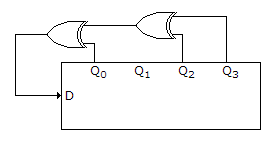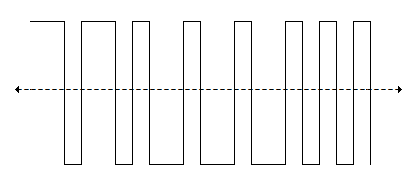# Electronics and Communication Engineering - Exam Questions Papers

26.

A 4 bit shift register is initialized to value 1000 for (Q3, Q2, Q1, Q0). The D input is derived from Q0, Q2 and Q3 through two XOR gates as shown in figure. The pattern 1000 will appear atA. 3rd pulse B. 7th pulse C. 6th pulse D. 4th pulse

Explanation:

No answer description available for this question. Let us discuss.

27.

Assertion (A): Capacitance of a solid conducting spherical body of radius 'a' is given by 4 pε0a in free space.

Reason (R): Δ x H = jωεE + J.

 A. Both A and R are individually true and R is the correct explanation of A B. Both A and R are individually true but R is not the correct explanation of A C. A is true but R is false D. A is false but R is true

Explanation:

No answer description available for this question. Let us discuss.

28.

Choose the correct statement/statements :

 A. In case of an antenna, the antenna resistance and loss resistance are not two different quantities. B. The loss resistance includes loss by eddy currents, improper earth connections, insulation leakages, etc., but not I2R losses. C. Radiation resistance varies directly as square-root of frequency D. None

Explanation:

No answer description available for this question. Let us discuss.

29.

The PCM signal shown below uses amplitude level of +1 volt and -1 volt to represent binary symbol 0 and 1 respectively. Each code word consists of 4 bits. The sampled version of analog signal from which this PCM signal is derived isA. EB596 B. CD231 C. DA495 D. 25B6A

Explanation:

No answer description available for this question. Let us discuss.

30.

Consider a situation where number of minterms with even number of 0's are same as number of minterms with odd number of 1's. Then minterms with even number of 0's and minterms with odd number of 1's are __________ to each other then number of variables is __________ .

 A. equivalent, even B. complement, odd C. complement, even D. none of these